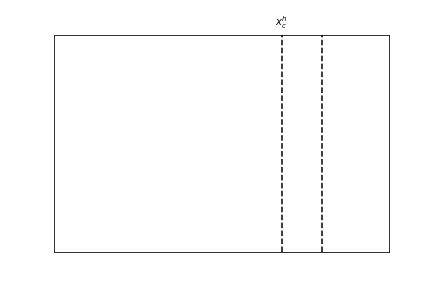# [SOLVED] Weird truncation of minor xticks

I have an odd problem where I am specifying two horizontal points where I would like minor xticks and for some reason, the last point is being truncated.

``````import matplotlib.pyplot as plt

x_c_h = 17.
x_c_l = 20.

ax = plt.axes()

plt.axvline(x_c_h,color='k',linestyle='--')
plt.axvline(x_c_l,color='k',linestyle='--')

ax.set_xticks([x_c_h,x_c_l,], minor=True)
ax.set_xticklabels(['\$x_c^h\$', '\$x_c^l\$'], minor=True)
ax.tick_params('x', which='minor', top=True, length=0,
labeltop=True, labelbottom=False)

ax.tick_params('x', which='major', labelleft=False,
labelbottom=False, length=0)
ax.tick_params('y', which='major', labelleft=False,
labelbottom=False, length=0)

plt.xlim(0, 25)
````````````ax.get_xticks(minor=True)
[17.0]

[tickl for tickl in ax.get_xminorticklabels()]
[Text(17.0, 1, '\$x_c^h\$')]
``````

This also seems to be a problem where I have more minor xticks where x_c_l is the final item in the list.

Any suggestions for fixing this? I’m on `matplotlib` version 3.2.2.

Thanks!

This seems to be a very weird `matplotlib` bug. If I change `x_c_l=20.01`, the problem disappears.

Minor ticks at positions of major ticks are removed by default. You can prevent that by `ax.xaxis.set_remove_overlapping_locs(False)` . However, the major and minor labels are then drawn on top of each other.

Thanks! It’s an illustrative figure so I suppressed the major ticks and found it completely baffling.